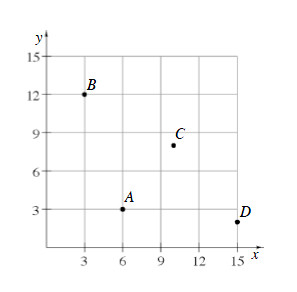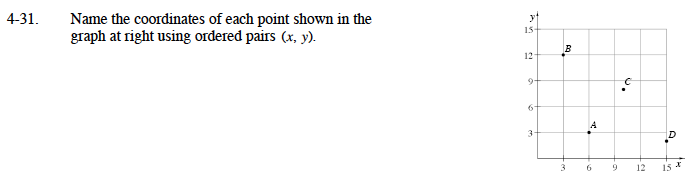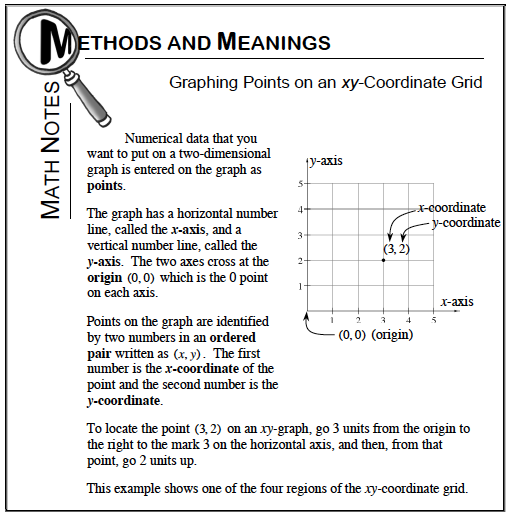### Home > MC1 > Chapter 4 > Lesson 4.1.3 > Problem4-31

4-31.

. Name the coordinates of each point shown in the graph below using ordered pairs ( x, y). Homework Help ✎Refer to the Math Notes box from Lesson 4.1.2 below to review ordered pairs.A = (6, 3) and B = (3, 12). Were you able to record the ordered pairs for C and D?